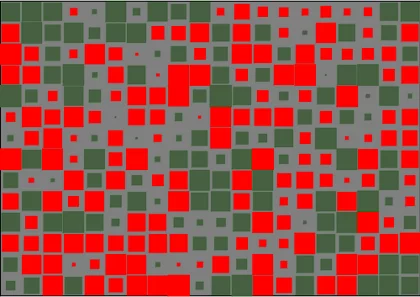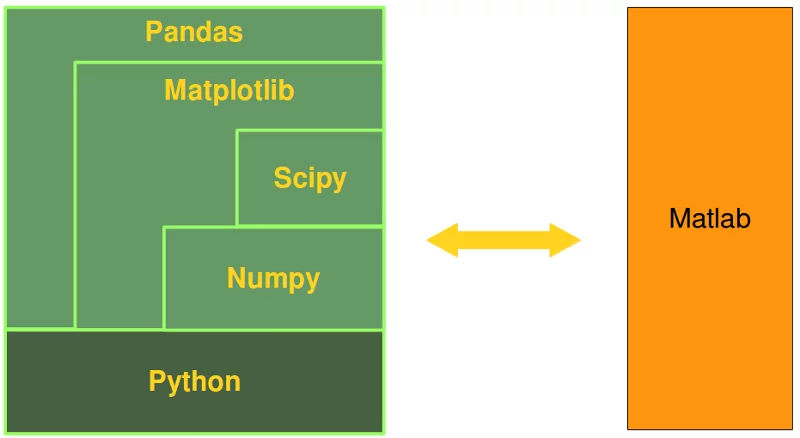# Contains SciPy NumPy

## Python courseNumPy is an acronym for "Numerical Python" (English: "Numeric Python" or "Numerical Python"). This is an extension module for Python, most of which is written in C.

This ensures that the compiled mathematical and numerical functions and functionalities guarantee the highest possible execution speed.

In addition, NumPy enriches the Python programming language with powerful data structures for efficient computing with large arrays and matrices.

The implementation even targets extremely large ("big data") matrices and arrays. The module also offers a huge number of high quality math functions to work with these matrices and arrays.

SciPy (Scientific Python) is often mentioned in the same breath as NumPy. SciPy extends the capabilities of NumPy with other useful functions, such as minimization, regression, Fourier transform and many others.

Both NumPy and SciPy are usually not installed in a standard Python installation. NumPy, as well as all the other modules mentioned, are part of the Anaconda distribution.

However, if you want to install NumPy manually, you should make sure that it is installed first, i.e. before SciPy. NumPy can be downloaded from the following website:

http://www.numpy.org

(Comment: The diagram in the picture on the right is a graphic visualization of a matrix with 14 rows and 20 columns. It is a so-called Hinton diagram. The size of a square within this diagram corresponds to the size of the corresponding value in the The color determines whether it is a positive or negative value. In our example: The color red denotes the negative values ​​and the color green denotes the positive values.)

NumPy is based on two previous Python modules that dealt with arrays. One of these is Numeric. Numeric, like NumPy, is a Python module for powerful numerical computation, but it is obsolete today. Another NumPy predecessor is Numarray, which is a complete overhaul of Numeric, but that module is also out of date today. NumPy is the amalgamation of these two, i.e. it is built on the code of Numeric and the functionalities of Numarray.

### The Python alternative to MATLAB

Python in combination with NumPy, SciPy, Matplotlib and Pandas can in principle be used as a full replacement for MATLAB. Python and its modules are free software ("free software" or "open source"), free in the sense of "freedom" and not "free" beer, even if Python is free.

Although a huge number of additional toolboxes are available for MATLAB, Python in conjunction with the modules mentioned above has the advantage that Python is the more modern and comprehensive programming language.

SciPy adds more MATLAB-like functionality to Python. The Matplotlib module offers the necessary plot functions. The youngest member of this family of modules is pandas. Pandas are ideal for working with tabular data, as is known from spreadsheet programs such as Excel.